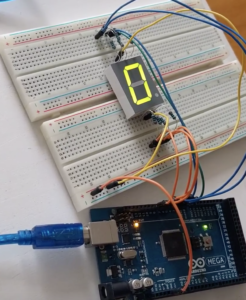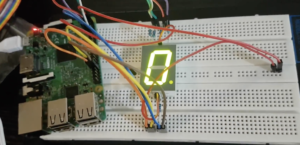# Raspberry Pi Servo Java Duke RobotWhat’s up?
2020/10/12

This post is to show how I created a Java Duke Servo demo with a Raspberry Pi. This is another blog about Java on Raspberry Pi.

Interfacing a Servo Motor with Raspberry Pi is an interesting topic as Servo Motors are the main components of a Robot and with the help of Raspberry Pi, you can have endless opportunities in Robotics.

Because today we celebrate children’s day in Brazil, I came with the idea to show this simple robot example using a servo motor and a Raspberry Pi. And nothing better than a Java Duke servo demo to show Java on Raspberry Pi.

## Components

• SG90 Micro-servo motor
• Jumper wires (generic)
• Raspberry Pi 3b or Arduino

## Idea

Using a cardboard and Duke image to create a servo demo where Duke moves his arm.I used this image to create my demo.

## Control a Servo Motor

To control a Servo Motor, you need to use a technique called Pulse Width Modulation or PWM. In PWM technique, you will be sending a pulse of variable width and the position of the Servo Motor’s shaft will be set by the width or length of the Pulse.

Nice “Pulse Width Modulation” explanation here

The frequency of the PWM signal is a fixed value and is dependent on the type of Servo Motor.
At 50Hz i.e. a period of 20ms, the minimum pulse width is 1ms and the maximum pulse width is 2ms. Most servo motors can have a swept area of 180 degrees i.e. 90 degrees on either side of the neutral position.

When the pulse width of the PWM Signal is 1ms, the position of the servo is the left. The Duty Cycle of this position is (1ms/20ms) x 100 = 5%.

Similarly, for pulse widths of 1.5ms and 2ms, the position of the servo is middle (with a duty cycle of 7.5%) and far right (with a duty cycle of 10%).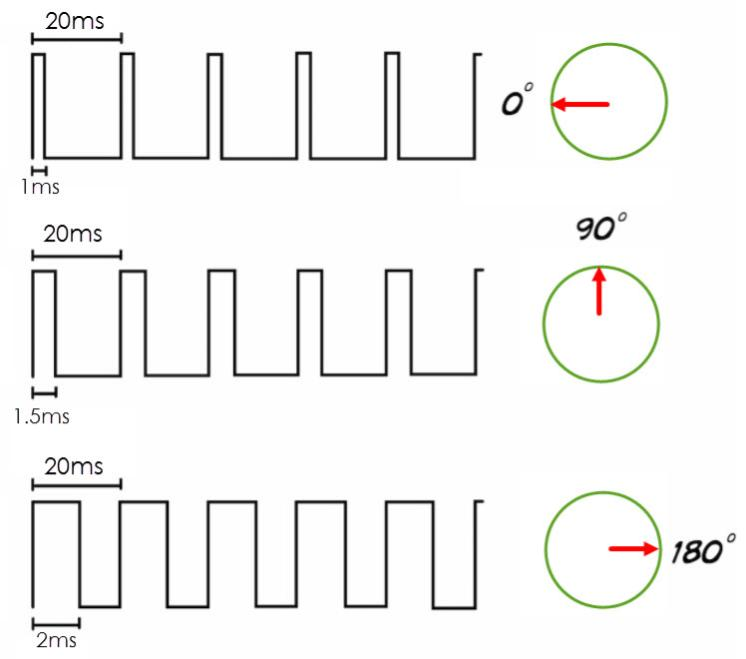• so for 50hz, one frequency is 20ms
• duty cycle for 0 degree = (1/20)*100 = 5%
• duty cycle for 90 degree = (1.5/20)*100 = 7.5%
• duty cycle for 180 degree = (2/20)*100 = 10%

Note: Be aware here that you need to test and check your servo. You might need to calibrate this number for you.

## Schematics

I’m using the SG90 servo motor and you can check the Datasheet here.

The Tower Pro SG90 Servo Motor Consists of three Pins: PWM (Orange or Yellow), VCC (Red), and GND (Brown). The VCC and GND pins must be connected to +5V and GND of the power supply.

PWM or Signal Pin of the Servo Motor must be connected to the PWM Output of the Controller (Raspberry Pi). Based on the width of the Pulses from the PWM signal, the angle of the Servo Motor’s shaft will vary.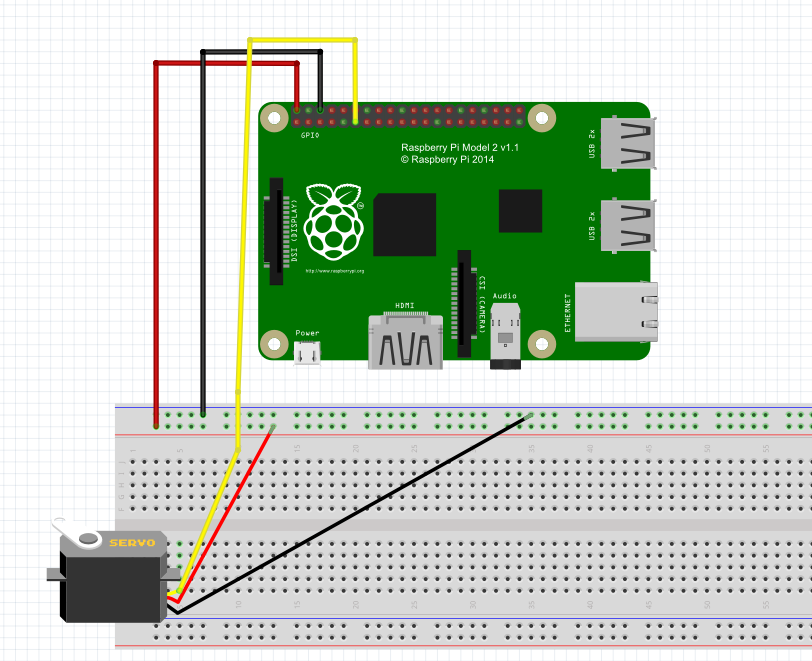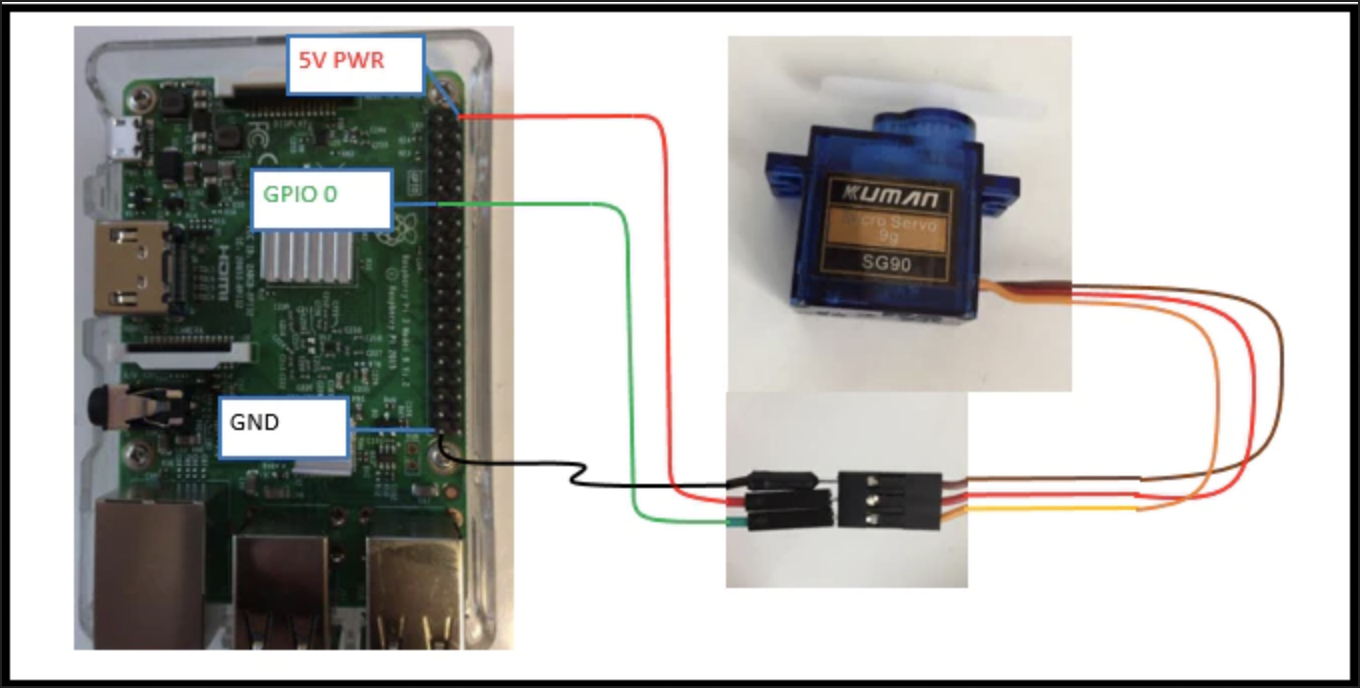1ms pulse for 0 degree (LEFT)
1.5ms pulse for 90 degree (MIDDLE)
2ms pulse for 180 degree (RIGHT)

## PI4J

 123456789101112131415161718192021222324252627282930313233343536373839404142434445464748 import com.pi4j.io.gpio.GpioController; import com.pi4j.io.gpio.GpioFactory; import com.pi4j.io.gpio.GpioPinPwmOutput; import com.pi4j.io.gpio.Pin; import com.pi4j.io.gpio.RaspiPin; import com.pi4j.util.CommandArgumentParser; public class TestPwmServoMotor {     public static void main(String[] args) throws InterruptedException {         pwm(args);     }     /**      * @param args      * @throws InterruptedException      */     public static void pwm(String[] args) throws InterruptedException{         final GpioController gpio = GpioFactory.getInstance();         Pin pin = CommandArgumentParser.getPin(         RaspiPin.class,             RaspiPin.GPIO_00,           args);                     GpioPinPwmOutput pwm = gpio.provisionSoftPwmOutputPin(pin);         pwm.setPwmRange(100);         int sleep = 1000;         for(int i = 0 ;i<10;i++){              pwm.setPwm(25);             System.out.println("PWM rate is: " + pwm.getPwm());             Thread.sleep(sleep);             pwm.setPwm(15);             System.out.println("PWM rate is: " + pwm.getPwm());             Thread.sleep(sleep);             pwm.setPwm(6);             System.out.println("PWM rate is: " + pwm.getPwm());             Thread.sleep(sleep);         }         gpio.shutdown();                 System.out.println("pwm end");     } }

## Python

 12345678910111213141516171819202122232425 import RPi.GPIO as GPIO import time control = [5,5.5,6,6.5,7,7.5,8,8.5,9,9.5,10] servo = 22 GPIO.setmode(GPIO.BOARD) GPIO.setup(servo,GPIO.OUT) p=GPIO.PWM(servo,50) p.start(2.5) try:        while True:            for x in range(11):              p.ChangeDutyCycle(control[x])              time.sleep(0.03)              print x                        for x in range(9,0,-1):              p.ChangeDutyCycle(control[x])              time.sleep(0.03)              print x             except KeyboardInterrupt:     GPIO.cleanup()

## Arduino

And of course, you can do this demo with an Arduino as well.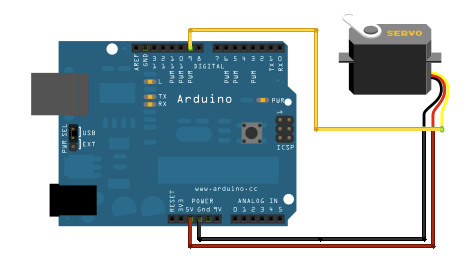1234567891011121314151617181920212223242526 #include Servo servo void setup() {   servo.attach(9);   } void loop() {   servo.write(0);   delay(500);   delay(10000);   servo.write(90);   delay(500);   delay(10000);   servo.write(180);   delay(500);   delay(10000);   servo.write(90);   delay(500);   delay(10000);   servo.write(0);   delay(500);   delay(10000); }

## Results

The next steps are to create a code that transforms text into the flag language

https://pi4j.com/1.2/index.html

https://github.com/Pi4J/pi4j

http://www.ee.ic.ac.uk/pcheung/teaching/DE1_EE/stores/sg90_datasheet.pdf

https://en.wikipedia.org/wiki/Pulse-width_modulation

https://www.electronics-tutorials.ws/blog/pulse-width-modulation.html

https://en.wikipedia.org/wiki/Flag_semaphore

# 7 Segment Display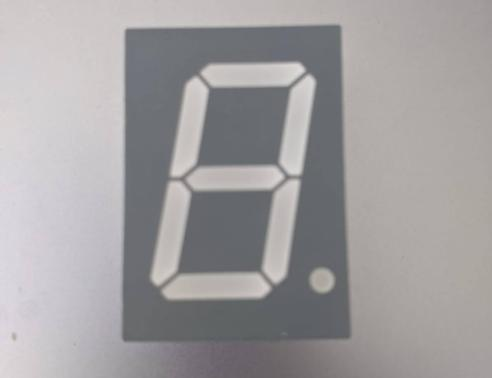What’s the crack jack?
2020/09/20

1. 7-Segment Display
2. Cathode vs Anode
3. Components
4. Schematics
5. Code
6. PI4J

## 1. 7-Segment Display

The 7-segment display, also written as “seven segment display”, consists of seven LEDs (hence its name) arranged in a rectangular fashion as shown. Each of the seven LEDs is called a segment because when illuminated the segment forms part of a numerical digit (both Decimal and Hex) to be displayed. An additional 8th LED is sometimes used within the same package thus allowing the indication of a decimal point (DP).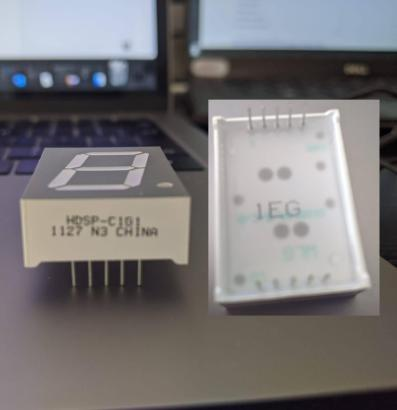A 7-segment is a packaged set of 8 LEDs (7 number-segments & 1 decimal point).

I have the “hdsp-c1g1” model and it is a Common-Anode.

## 2. Cathode vs Anode

Common anode means that the anode (positive) side of all of the LEDs is electrically connected at one pin, and each LED cathode has its own pin. So turning on any particular segment will involve running a current from this common anode (positive) pin to the particular cathode (negative) pin for the desired segment.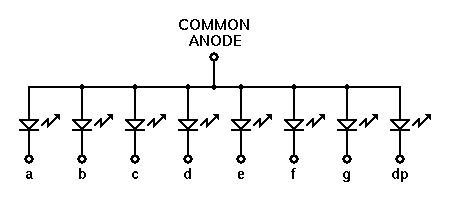Common cathode means that the cathodes of all of the LEDs are common and connected to a single pin. The anode for each LED has its own pin. So driving one of these means running a current from the particular anode (positive) pin for the desired segment to the common cathode pin.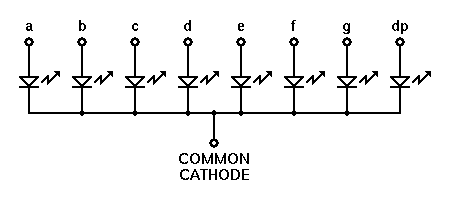The way that you are diving the 7-segment involves using a separate pin to drive each segment, so you use 8 pins of the Arduino to drive the display.

## 3. Components

• 1x Arduino
• 1x Raspberry Pi
• 1x Common-Anode 7-segment display
• 8x Resistor 221 ohm
• 10x Jumper wires (generic)

## 4. Schematics

Common-Anode 7-segment display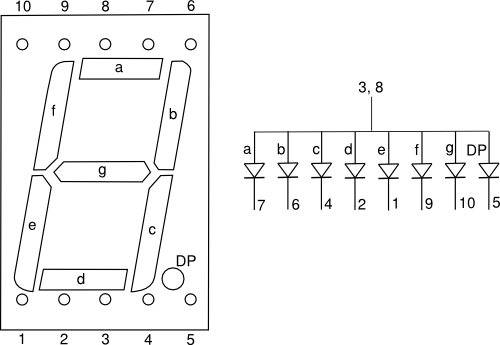My 7-segment display has its common pins (COM) connected to the +5V pin.
If a pin turns off, the corresponding segment will turn on. It’s because when the pin is on, the voltage difference between the pin and the +5V is 0. It is the same if the +5V was replaced by 0V. We can’t connect the COM to the GND because the LEDs are like diodes so they wouldn’t light up at all.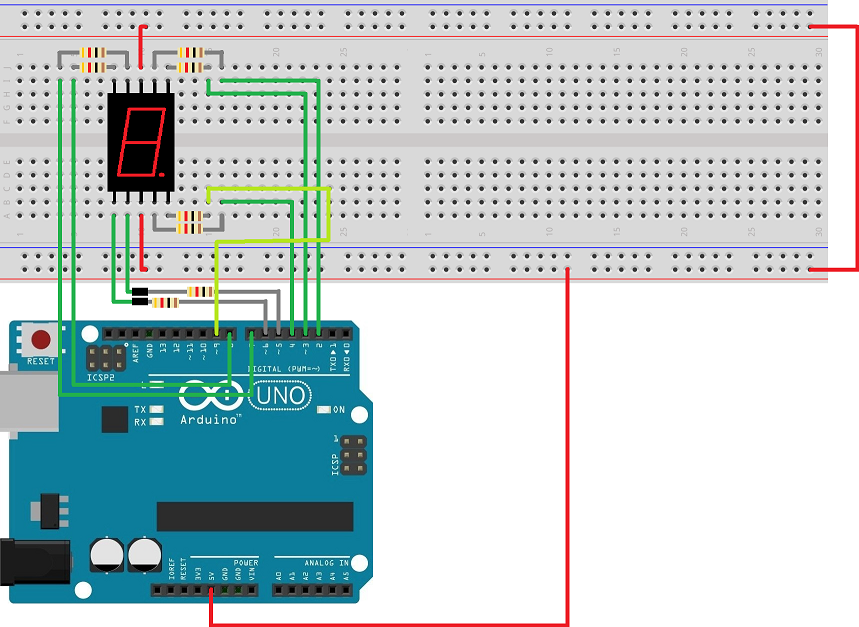For Raspberry PI you need to check the GPIO numbers. Note that the pin number is not the same thing as the GPIO number.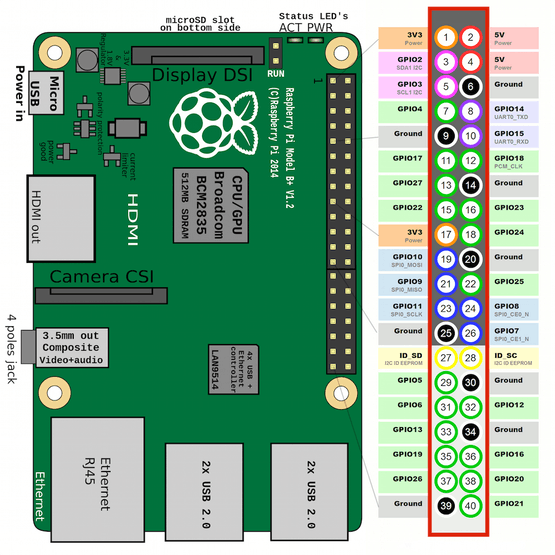For my example here I create a table to map: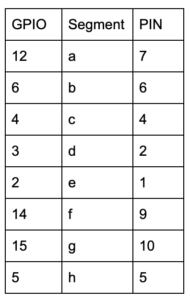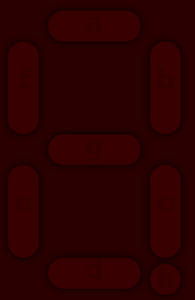## 5. Code

Arduino

 123456789101112131415161718192021222324252627282930313233343536373839404142434445464748495051525354555657585960616263646566676869707172737475767778798081828384858687888990919293949596979899100101102103104105106107108109110111112113114115116117118119120121122123124125126127128129130131132133134135136137138139140141142143144145146147148149150151152153154155156157158159160161162163164165166167168169170171172 void setup() {   init();   /*your setup code here*/ } void loop() {   /*    * your loop code here    *    * use display(num, dp) to display num (0123456789ABCDEF)    * and the dot (if dp).    *    * you can turn off all the leds by writing display().    */ } void init() {   pinMode(2, OUTPUT);   pinMode(3, OUTPUT);   pinMode(4, OUTPUT);   pinMode(5, OUTPUT);   pinMode(6, OUTPUT);   pinMode(7, OUTPUT);   pinMode(8, OUTPUT);   pinMode(9, OUTPUT); } void display(int n=-1, boolean dp) {   digitalWrite(9, !dp);   if(num == 0)   {     digitalWrite(2, 0);     digitalWrite(3, 0);     digitalWrite(4, 0);     digitalWrite(5, 0);     digitalWrite(6, 0);     digitalWrite(7, 0);     digitalWrite(8, 1);   } else if(num == 1) {     digitalWrite(2, 1);     digitalWrite(3, 0);     digitalWrite(4, 0);     digitalWrite(5, 1);     digitalWrite(6, 1);     digitalWrite(7, 1);     digitalWrite(8, 1);   } else if(num == 2) {     digitalWrite(2, 0);     digitalWrite(3, 0);     digitalWrite(4, 1);     digitalWrite(5, 0);     digitalWrite(6, 0);     digitalWrite(7, 1);     digitalWrite(8, 0);   } else if(num == 3) {     digitalWrite(2, 0);     digitalWrite(3, 0);     digitalWrite(4, 0);     digitalWrite(5, 0);     digitalWrite(6, 1);     digitalWrite(7, 1);     digitalWrite(8, 0);   } else if(num == 4) {     digitalWrite(2, 1);     digitalWrite(3, 0);     digitalWrite(4, 0);     digitalWrite(5, 1);     digitalWrite(6, 1);     digitalWrite(7, 0);     digitalWrite(8, 0);   } else if(num == 5) {     digitalWrite(2, 0);     digitalWrite(3, 1);     digitalWrite(4, 0);     digitalWrite(5, 0);     digitalWrite(6, 1);     digitalWrite(7, 0);     digitalWrite(8, 0);   } else if(num == 6) {     digitalWrite(2, 0);     digitalWrite(3, 1);     digitalWrite(4, 0);     digitalWrite(5, 0);     digitalWrite(6, 0);     digitalWrite(7, 0);     digitalWrite(8, 0);   } else if(num == 7) {     digitalWrite(2, 0);     digitalWrite(3, 0);     digitalWrite(4, 0);     digitalWrite(5, 1);     digitalWrite(6, 1);     digitalWrite(7, 1);     digitalWrite(8, 1);   } else if(num == 8) {     digitalWrite(2, 0);     digitalWrite(3, 0);     digitalWrite(4, 0);     digitalWrite(5, 0);     digitalWrite(6, 0);     digitalWrite(7, 0);     digitalWrite(8, 0);   } else if(num == 9) {     digitalWrite(2, 0);     digitalWrite(3, 0);     digitalWrite(4, 0);     digitalWrite(5, 0);     digitalWrite(6, 1);     digitalWrite(7, 0);     digitalWrite(8, 0);   } else if(num == 10) {     digitalWrite(2, 0);     digitalWrite(3, 0);     digitalWrite(4, 0);     digitalWrite(5, 1);     digitalWrite(6, 0);     digitalWrite(7, 0);     digitalWrite(8, 0);   } else if(num == 11) {     digitalWrite(2, 1);     digitalWrite(3, 1);     digitalWrite(4, 0);     digitalWrite(5, 0);     digitalWrite(6, 0);     digitalWrite(7, 0);     digitalWrite(8, 0);   } else if(num == 12) {     digitalWrite(2, 0);     digitalWrite(3, 1);     digitalWrite(4, 1);     digitalWrite(5, 0);     digitalWrite(6, 0);     digitalWrite(7, 0);     digitalWrite(8, 1);   } else if(num == 13) {     digitalWrite(2, 1);     digitalWrite(3, 0);     digitalWrite(4, 0);     digitalWrite(5, 0);     digitalWrite(6, 0);     digitalWrite(7, 1);     digitalWrite(8, 0);   } else if(num == 14) {     digitalWrite(2, 0);     digitalWrite(3, 1);     digitalWrite(4, 1);     digitalWrite(5, 0);     digitalWrite(6, 0);     digitalWrite(7, 0);     digitalWrite(8, 0);   } else if(num == 15) {     digitalWrite(2, 0);     digitalWrite(3, 1);     digitalWrite(4, 1);     digitalWrite(5, 1);     digitalWrite(6, 0);     digitalWrite(7, 0);     digitalWrite(8, 0);   } else {     digitalWrite(2, 1);     digitalWrite(3, 1);     digitalWrite(4, 1);     digitalWrite(5, 1);     digitalWrite(6, 1);     digitalWrite(7, 1);     digitalWrite(8, 1);   } }

Raspberry PI – Python

 123456789101112131415161718192021222324252627282930313233343536373839404142434445464748495051525354555657585960616263646566676869707172737475767778798081828384858687888990919293949596979899100101102103104105106107108109110111112113114115116117118119120121122123 from gpiozero import LED from time import sleep pin01 = LED(2) pin02 = LED(3) pin04 = LED(4) pin05 = LED(5) pin06 = LED(6) pin07 = LED(12) pin09 = LED(14) pin10 = LED(15) def clear():     pin01.on()     pin02.on()     pin04.on()     pin05.on()     pin06.on()     pin07.on()     pin09.on()     pin10.on() def display(number):     if number == 0:         pin01.off()         pin02.off()         pin04.off()         pin05.on()         pin06.off()         pin07.off()         pin09.off()         pin10.on()     if number == 1:         pin01.on()         pin02.on()         pin04.off()         pin05.on()         pin06.off()         pin07.on()         pin09.on()         pin10.on()     if number == 2:         pin01.off()         pin02.off()         pin04.on()         pin05.on()         pin06.off()         pin07.off()         pin09.on()         pin10.off()     if number == 3:         pin01.on()         pin02.off()         pin04.off()         pin05.on()         pin06.off()         pin07.off()         pin09.on()         pin10.off()     if number == 4:         pin01.on()         pin02.on()         pin04.off()         pin05.on()         pin06.off()         pin07.on()         pin09.off()         pin10.off()     if number == 5:         pin01.on()         pin02.off()         pin04.off()         pin05.on()         pin06.on()         pin07.off()         pin09.off()         pin10.off()     if number == 6:         pin01.off()         pin02.off()         pin04.off()         pin05.off()         pin06.on()         pin07.off()         pin09.off()         pin10.off()     if number == 7:         pin01.on()         pin02.on()         pin04.off()         pin05.on()         pin06.off()         pin07.off()         pin09.on()         pin10.on()     if number == 8:         pin01.off()         pin02.off()         pin04.off()         pin05.on()         pin06.off()         pin07.off()         pin09.off()         pin10.off()     if number == 9:         pin01.on()         pin02.on()         pin04.off()         pin05.off()         pin06.off()         pin07.off()         pin09.off()         pin10.off() clear() sleep(1) while True:     for x in range(10):         display(x)         sleep(1)

## 6. PI4J

PI4J provides a friendly object-oriented I/O API and implementation libraries for Java programmers to access the full I/O capabilities of the Raspberry Pi platform. This project abstracts the low-level native integration and interrupts monitoring to enable Java programmers to focus on implementing their application business logic.

Note: Pi4J (by default) uses an abstract pin numbering scheme to help insulate software from hardware changes.Pi4J implements the same pin number scheme as the Wiring Pi

http://wiringpi.com/pins/

This is the Number for the PI 3 B model.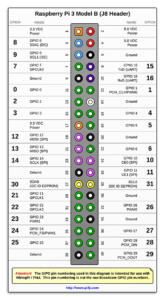Because the PI4J use a different GPIO number I add a new column in my table;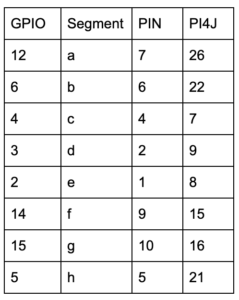Raspberry PI – Java – Pi4j

 123456789101112131415161718192021222324252627282930313233343536373839404142434445464748495051525354555657585960616263646566676869707172737475767778798081828384858687888990919293949596979899100101102103104105106107108109110111112113114115116117118119120121122123124125126127128129130131132133134135136137138139140141142143144145146147148149150151152153154155156157158159160161162 package led.example; import com.pi4j.io.gpio.GpioController; import com.pi4j.io.gpio.GpioFactory; import com.pi4j.io.gpio.GpioPinDigitalOutput; import com.pi4j.io.gpio.PinState; import com.pi4j.io.gpio.RaspiPin; public class SevenSegmentsDisplay {     static GpioPinDigitalOutput pin01 = null;     static GpioPinDigitalOutput pin02 = null;     static GpioPinDigitalOutput pin04 = null;     static GpioPinDigitalOutput pin05 = null;     static GpioPinDigitalOutput pin06 = null;     static GpioPinDigitalOutput pin07 = null;     static GpioPinDigitalOutput pin09 = null;     static GpioPinDigitalOutput pin10 = null;     public static void display(int number) {         if(number == 0) {             pin01.low();             pin02.low();             pin04.low();             pin05.high();             pin06.low();             pin07.low();             pin09.low();             pin10.high();         }         if(number == 1) {             pin01.high();             pin02.high();             pin04.low();             pin05.high();             pin06.low();             pin07.high();             pin09.high();             pin10.high();         }         if(number == 2) {             pin01.low();             pin02.low();             pin04.high();             pin05.high();             pin06.low();             pin07.low();             pin09.high();             pin10.low();         }         if(number == 3) {             pin01.high();             pin02.low();             pin04.low();             pin05.high();             pin06.low();             pin07.low();             pin09.high();             pin10.low();         }         if(number == 4) {             pin01.high();             pin02.high();             pin04.low();             pin05.high();             pin06.low();             pin07.high();             pin09.low();             pin10.low();         }         if(number == 5) {             pin01.high();             pin02.low();             pin04.low();             pin05.high();             pin06.high();             pin07.low();             pin09.low();             pin10.low();         }         if(number == 6) {             pin01.low();             pin02.low();             pin04.low();             pin05.low();             pin06.high();             pin07.low();             pin09.low();             pin10.low();         }         if(number == 7) {             pin01.high();             pin02.high();             pin04.low();             pin05.high();             pin06.low();             pin07.low();             pin09.high();             pin10.high();         }         if(number == 8) {             pin01.low();             pin02.low();             pin04.low();             pin05.high();             pin06.low();             pin07.low();             pin09.low();             pin10.low();         }         if(number == 9) {             pin01.high();             pin02.low();             pin04.low();             pin05.low();             pin06.low();             pin07.low();             pin09.low();             pin10.low();         }     }     public static void main(String[] args) {         final GpioController gpio = GpioFactory.getInstance();         pin01 = gpio.provisionDigitalOutputPin(RaspiPin.GPIO_08);         pin02 = gpio.provisionDigitalOutputPin(RaspiPin.GPIO_09);         pin04 = gpio.provisionDigitalOutputPin(RaspiPin.GPIO_07);         pin05 = gpio.provisionDigitalOutputPin(RaspiPin.GPIO_21);         pin06 = gpio.provisionDigitalOutputPin(RaspiPin.GPIO_22);         pin07 = gpio.provisionDigitalOutputPin(RaspiPin.GPIO_26);         pin09 = gpio.provisionDigitalOutputPin(RaspiPin.GPIO_15);         pin10 = gpio.provisionDigitalOutputPin(RaspiPin.GPIO_16);         pin01.setShutdownOptions(true, PinState.HIGH);         pin02.setShutdownOptions(true, PinState.HIGH);         pin04.setShutdownOptions(true, PinState.HIGH);         pin05.setShutdownOptions(true, PinState.HIGH);         pin06.setShutdownOptions(true, PinState.HIGH);         pin07.setShutdownOptions(true, PinState.HIGH);         pin09.setShutdownOptions(true, PinState.HIGH);         pin10.setShutdownOptions(true, PinState.HIGH);         try {             while(true) {                 for (int i = 0; i < 10; i++) {                     display(i);                     System.out.println("Number " + i);                     Thread.sleep(2000);                 }             }         } catch (InterruptedException e) {             e.printStackTrace();         } finally {             gpio.shutdown();         }     } }

Results